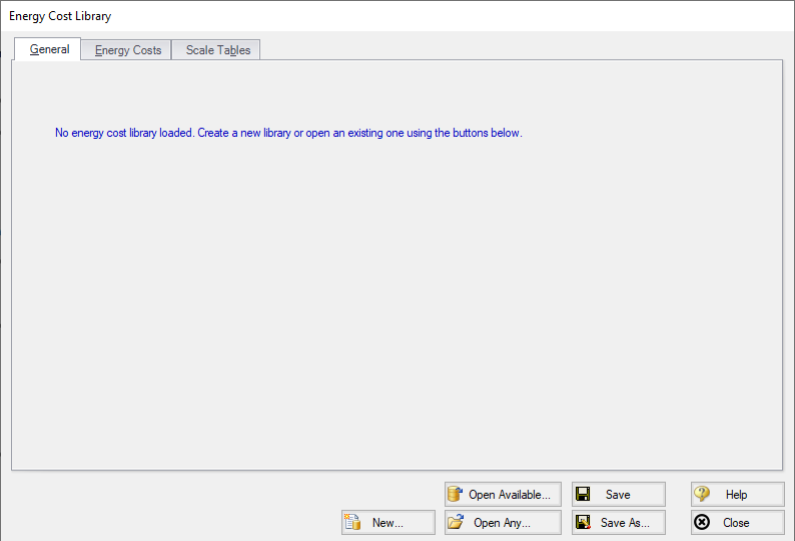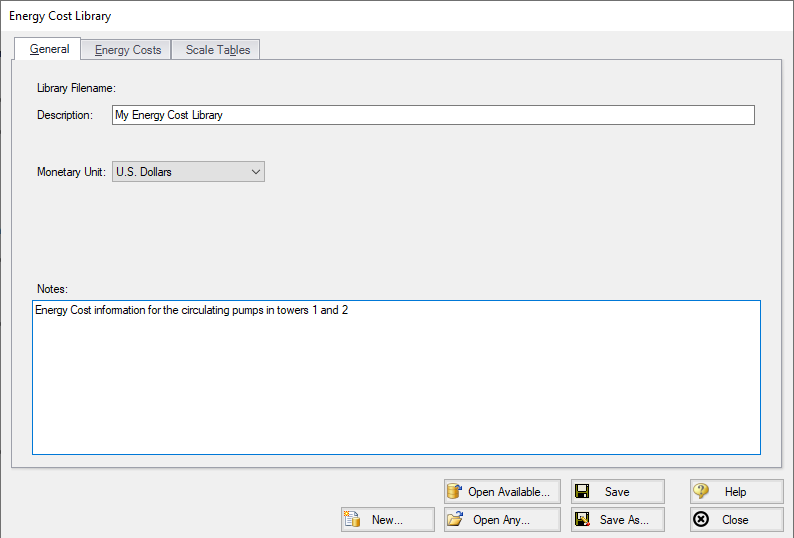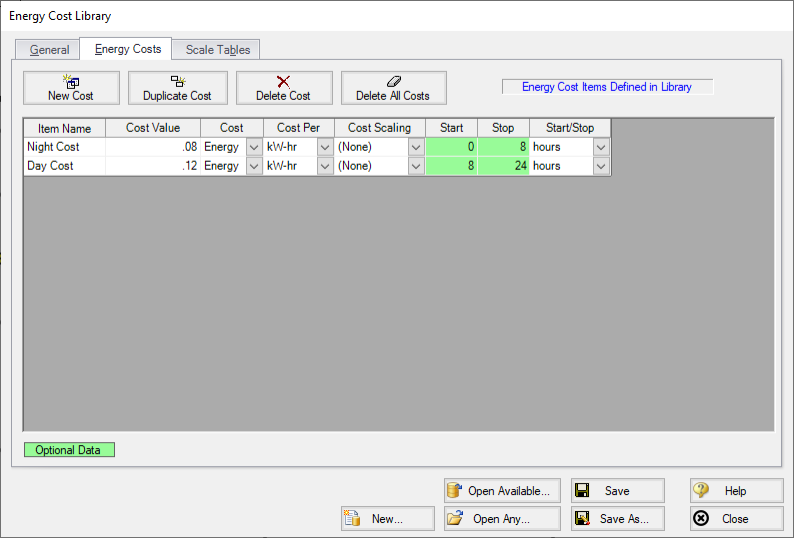## Energy Cost Library

Energy Cost Libraries allow users to input time varying energy costs. These energy costs can then be assigned to pumps, so that pump operation/energy costs can be calculated.

Creating an Energy Cost Library

When opening the Energy Cost Library window from the Library menu, you will be greeted with Figure 1. An Energy Cost Library either needs to be created or opened.Figure 1: Energy Cost Library window

To create a new Energy Cost Library, click the New button. Give the Library a Description and, optionally, write some notes for it. Select an alternate Monetary Unit if desired.Figure 2: New Energy Cost Library

Finally, save the library by clicking Save As. A prompt will appear asking for a name for the *.ecd file. AFT Fathom will also prompt if the library should be connected and available - typically this is desired so the library can be used in the currently open model.

Defining Energy Costs

Now that an Energy Cost Library exists, some costs should be added to it. Select the Energy Costs tab.Figure 3: Energy Costs

Select New Cost to add costs to the library. In Figure 3, costs have been added to represent a varying electricity cost over night and day. If the Cost Time Period exceeds the time defined in the Energy Cost Library, the pattern will start over at zero. That is, for the above example if the Cost Time Period was one year the defined 24 hour cost cycle would repeat 365 times.

The Cost Scaling column allows a Scale Table to be applied to a cost, as discussed below.

Scale Tables

You can add a time-based multiplier to an Energy Cost Library on top of the normal Energy Costs if desired. The Scale Table can be used, for example, to represent the increasing cost of electricity year by year. See Scale Tables.

For energy cost libraries, the Scale Tables are limited to entering a multiplier over time.

Library Connection

Creating a new Energy Cost Library will prompt connecting it automatically. If for whatever reason an existing Library is not connected in can be manually connected with the Library Manager.